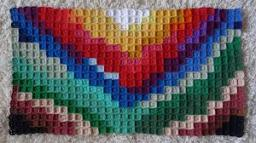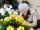# Paper squares

The paper rectangle measuring 69 cm and 46 cm should be cut into as many squares as possible. Calculate the lengths of squares and their number.

x =  23 cm
n =  6

### Step-by-step explanation:Did you find an error or inaccuracy? Feel free to write us. Thank you!Tips to related online calculators
Do you want to calculate greatest common divisor two or more numbers?

#### You need to know the following knowledge to solve this word math problem:

We encourage you to watch this tutorial video on this math problem:

## Related math problems and questions:

• PlumberThe plumber had to cut the metal strip with dimensions 380 cm and 60 cm to the largest squares to no waste. Calculate the length of the sides of a square. How many squares cut it?
• PexesoDrawing paper has dimensions of 60cm and 840mm. Pupils have to divide it into squares so that they can make a pexeso. What dimension must squares have if their side is to be larger than 3cm and less than 10cm?
• Largest squaresHow many of the largest square sheets did the plumber cut the honeycomb from 16 dm and 96 dm?
• Glass panelA rectangular glass panel with dimensions of 72 cm and 96 cm will cut the glazier on the largest square possible. What is the length of the side of each square? How many squares does the glazier cut?
• Gardens colonyGardens colony with dimensions of 180 m and 300 m are to be completely divided into the same large squares of the highest area. Calculate how many such squares can be obtained and determine the length of the square side.
• PegsFrom two sticks 240 cm and 210 cm long, it is necessary to cut the longest possible pegs for flowers so that no residues remain. How many pegs will it be?
• Square tilesThe room has dimensions of 12 meters and 5.6 meters. Determine the number of square tiles and their largest dimension to exactly cover the floor.
• TilesHall has dimensions 325 &time; 170 dm. What is the largest size of square tiles that can be entire hall tiled, and how many we need them?
• GardenThe garden has the shape of a rectangle measuring 19m20cm and 21m60cm. Mr. Novák will fence it. It wants the distance between adjacent pillars to be at least two meters and a maximum of three meters. He would also like the distances between the adjacent p
• CubesCarol with cut bar 12 cm x 12 cm x 135 cm to the cubes. Find the sum of all the surfaces of the resulting cubes.
• Common divisorsFind all common divisors of numbers 30 and 45.
• GroupsIn the 6th class there are 60 girls and 72 boys. We want to divide them into groups so that the number of girls and boys is the same. How many groups can you create? How many girls will be in the group?
• Cutting paperDivide a rectangular paper with dimensions 220mm and 308mm into squares of the same size so that they are as large as possible. Specify the length of the side of the square.
• FloristFlorist has 84 red and 48 white roses. How many same bouquets can he make from them if he must use all roses?
• SegmentsLine segments 62 cm and 2.2 dm long we divide into equal parts which lengths in centimeters is expressed integer. How many ways can we divide?
• Square gardensThe gardening colony with dimensions of 180 m and 300 m is to be completely divided into equally large square areas with the largest possible area. Calculate how many such square areas can be obtained and determine the side length of the square.
• RectanglesCalculate how many squares/rectangles of size 4×3 cm can be cut from a sheet of paper of 36 cm × 32 cm?